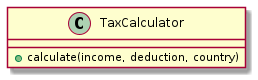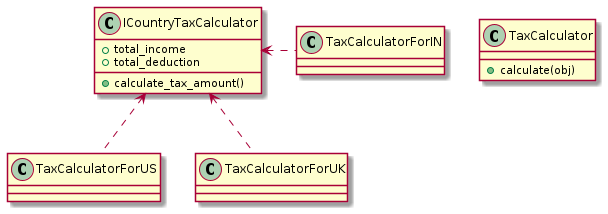# How to Write Clean Code (in Python) With SOLID Principles | Principle 2

Created on Dec 25, 2021
Updated on Jan 8, 2022

This is part 2 from the series of articles on the SOLID principles. If you missed the first part where we talked about the Single Responsibility Principle, please check it out and let me know in the comments if you have any questions.

So let’s dive into the second design principle: Open/Closed Principle (the ‘O’ in SOLID).

With illustration of how we can identify the Open-Closed Principle (OCP) implemented in Python. You’ll see the demonstration in a UML diagram to show the connections between the classes before and after refactoring. Will go through that through a real-world example.

Definition:

Software entities (modules, classes, functions, etc.) should be open for extension, but closed for modification.

The open/closed principle was first proposed by Bertrand Meyer, creator of the Eiffel programming language, and the idea of design by contract.

A unit of code can be considered “open for extension” when its behavior can be easily changed without modifying it. The fact that no actual modification is needed to change the behavior of a unit of code makes it “closed” for modification.

The purpose of this principle is to be able to extend the behavior of an entity without ever modifying its source code.

This happens when your objects are open to extension (using inheritance) but closed to alteration (by altering methods or changing values in an object).

## Example: Tax Calculator

Suppose you are developing a web application that includes an online tax calculator.

Users can visit a web page, specify their income and expense details, and calculate the tax payable using some mathematical calculation.

Considering this, you created a `TaxCalculator` class as shown below:TaxCalculator class. (Designed by Plantuml)

The `TaxCalculator` class has a single public method, `calculate()` , that accepts total income, total deduction, and country of the user.

Of course, a real-world tax calculator would do much more, but this simple design is sufficient for our example.

The `country` information is necessary because tax rules are different across different countries. The pseudo-code of the `calculate()` method is shown below:

``````def calculate(income, deduction, country):
# tax_amount variable is defined
# in each calculation
taxable_income = income - deduction
if country == "India":
# calculation here
elif country == "US":
# calculation here
elif country == "UK":
# calculation here
return tax_amount
``````

The `calculate()` method determines the taxable income by subtracting total deduction from total income.

Have you noticed the if conditions in the `calculate()` method? Condition after another to choose the right tax calculation based on the value of the `country` of the user as a parameter.

This branching logic is a good example of a violation of the Open/Closed Principle.

You might say, what’s the problem with that? Well, the problem is that if we add a new country, we have to modify the `calculate()` method because this method now considers only three countries.

Although when we think about scaling and users from several countries start using the web app, then there would be a problem.

When that happens, the `TaxCalculator` class needs to change to accommodate the new countries and their corresponding taxation rules. Thus, the current design violates OCP.

## How to spot OCP violations

To recognize if there is a violation of the open-closed principle, there is a list of symptoms that can be used to detect such violations:

• There are conditions to determine a strategy just like the if conditions in the `calculate()` method.
• Same variables or constants are used in conditions and recurring inside the same class or related classes.
• Hard-coded references to other classes are used inside the class.
• Objects are created inside the class.

These are all good reasons to adhere to the Open/Closed Principle.

### How to refactor to adhere to OCP

Now, let’s rectify the class design. Have a look at the UML diagram below:Applying OCP when calculating taxes. (Designed by Plantuml)

Note: In the figure above, the first compartment of the `ICountryTaxCalculator ` block indicates that it’s an interface, the second compartment contains a list of properties, and the third compartment contains a method.

That UML diagram is depicted as follows: Arrows with dotted lines, with the unfilled arrowhead, start from the classes (like `TaxCalculatorForIN ` , `TaxCalculatorForUS` , and ` TaxCalculatorForUK` ) that implement the `ICountryTaxCalculator` interface and point toward that interface being implemented.

The modified design has an abstraction in the form of the implemented interface. This interface contains two properties `total_income` and `total_deduction ` , and one method `calculate_tax_amount()` .

What’s changed already? The `TaxCalculator` no longer includes the tax calculation logic and is each tax logic is implemented in a separate class depending on the country.

This way, the logic of calculating taxes is wrapped in a separate unit.

Notice the change to the `calculate()` method of `TaxCalculator` . It now accepts a single parameter, `obj` , of type `ICountryTaxCalculator ` .

The pseudo-code for the modified `calculate()` method is shown below:

``````class TaxCalculator:
def calculate(self, obj: ICountryTaxCalculator):
tax_amount = 0
# some more logic here
tax_amount = obj.calculate_tax_amount();
return tax_amount
``````

As you can see, now the `calculate()` method doesn’t check for the country. The reason is that it receives an object as its parameter that implements the `ICountryTaxCalculator` interface. So, calling `calculate_tax_amount() ` returns the tax amount no matter which country the user belongs to.

Thus, the `TaxCalculator` class now conforms to OCP. If you need to calculate for a country not currently covered, all you need to do is to create another class that inherits from the `ICountryTaxCalculator` class and writes the tax calculation logic there.

`TaxCalculator` should be open for extending the functionality (by adding new country-specific classes that implement `ICountryTaxCalculator ` ), and meanwhile, it should also be closed for modification (you don’t need to change its source code).

``````from abc import ABC, abstractmethod

class ICountryTaxCalculator(ABC):
@abstractmethod
def calculate_tax_amount(self):
pass
``````

So that’s the `ICountryTaxCalculator` interface. An abstract class that has just one abstract method.

We now can implement three classes from that interface: `TaxCalculatorForUS ` , `TaxCalculatorForUK` , and ` TaxCalculatorForIN` .

Let’s see how we create these classes after `ICountryTaxCalculator` has been implemented.

``````class TaxCalculatorForUS(ICountryTaxCalculator):
def __init__(self, total_income, total_deduction):
self.total_income = total_income
self.total_deduction = total_deduction

def calculate_tax_amount(self):
taxable_income = self.total_income - self.total_deduction
return taxable_income * 30 / 100

class TaxCalculatorForUK(ICountryTaxCalculator):
def __init__(self, total_income, total_deduction):
self.total_income = total_income
self.total_deduction = total_deduction

def calculate_tax_amount(self):
taxable_income = self.total_income - self.total_deduction
return taxable_income * 35 / 100

class TaxCalculatorForIN(ICountryTaxCalculator):
def __init__(self, total_income, total_deduction):
self.total_income = total_income
self.total_deduction = total_deduction

def calculate_tax_amount(self):
taxable_income = self.total_income - self.total_deduction
return taxable_income * 20 / 100
``````

The `calculate_tax_amount()` method implemented by these classes finds taxable income by subtracting deductions from the income.

This value is treated as a taxable income, and a certain percentage of it (30%, 35%, and 20%, respectively) is returned to the caller as the tax amount.

Now add `TaxCalculator` class and modify it as shown below:

``````class TaxCalculator:
def calculate(self, ICountryTaxCalculator: obj):
tax_amount = obj.calculate_tax_amount();
# do something more if needed
return tax_amount
``````

The `calculate()` method accepts an object of a type that implements `ICountryTaxCalculator ` and invokes `calculate_tax_amount()` method. The tax amount is then returned to the caller.

Although not required in this example, you may do some extra processing in addition to calling `calculate_tax_amount()` .

## Final thoughts:

It is a simple fact that software systems evolve over time. New requirements must constantly be satisfied, and existing requirements must be changed according to customer needs or technology progress.

Applying the Open/Closed Principle is a good way to maintain any extension required for your codebase.

## Credit

• Beginning SOLID Principles and Design Patterns for ASP.NET Developers by Bipin Joshi